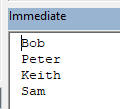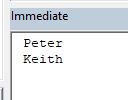# VBA Loop Through Array / For Each Item in Array

This tutorial will teach you how to loop through Arrays in VBA.

There are two primary ways to loop through Arrays using VBA:

• For Each Loop – The For Each Loop will loop through each item in the array.
• For Next Loop – The For Next Loop will loop through specified start and end positions of the array (We can use the UBound and LBound Functions to loop through the entire array).

## For Each Item in Array

The For Each Loop enables you to loop through each element of the array.

``````Sub LoopForArrayStatic()
'declare a variant array
Dim strNames(1 To 4) As String

'populate the array
strNames(1) = "Bob"
strNames(2) = "Peter"
strNames(3) = "Keith"
strNames(4) = "Sam"

'declare a variant to hold the array element
Dim item as variant

'loop through the entire array
For Each item in strNames
'show the element in the debug window.
Debug.Print item
Next item
End Sub``````

The above procedure will loop through all the names in the array.## For Next Loop

The For Next Loop will loop through each item at a specified start and end position of the array.

### Loop Through Part of Array

You can manually specify the start and end positions for your loop. This may be appropriate if you know your array size and/or you only want to loop through part of an array.

``````Sub LoopForNextStatic()
'declare a variant array
Dim strNames(1 To 4) As String
'populate the array
strNames(1) = "Bob"
strNames(2) = "Peter"
strNames(3) = "Keith"
strNames(4) = "Sam"
'declare an integer
Dim i As Integer
'loop from position 2 to position 3 of the array
For i = 2 To 3
'show the name in the immediate window
Debug.Print strNames(i)
Next i
End Sub``````

In the example above, we have looped through positions 2 and 3 of the array.  The immediate window would return the names as follows.### Loop Through Entire Array

Next, we will use the UBound and LBound Functions to loop through an entire array. This is extremely useful if the start and end positions of the array might change (ex. a Dynamic Array):

``````Sub LoopForNextDynamic()
'declare a variant array
Dim strNames() As String
'initialize the array
ReDim strNames(1 to 4)
'populate the array
strNames(1) = "Bob"
strNames(2) = "Peter"
strNames(3) = "Keith"
strNames(4) = "Sam"
'declare an integer
Dim i As Integer
'loop from the lower bound of the array to the upper bound of the array - the entire array
For i = LBound(strNames) To UBound(strNames)
'show the name in the immediate window
Debug.Print strNames(i)
Next i
End Sub``````

Stop searching for VBA code online. Learn more about AutoMacro - A VBA Code Builder that allows beginners to code procedures from scratch with minimal coding knowledge and with many time-saving features for all users!